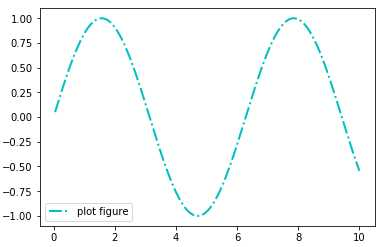# python可视化---legend()函数

loc：图例在图中的地理位置

```import matplotlib.pyplot as plt
import numpy as np

x = np.linspace(0.05, 10, 1000)
y = np.sin(x)

plt.plot(x, y, ls="-.", lw=2, c="c", label="plot figure")

plt.legend(loc="lower left")

plt.show()```python可视化---legend()函数

(0)
(0)